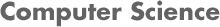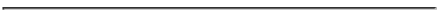### CS 548 KNOWLEDGE DISCOVERY AND DATA MINING - Spring 2014 Project 2: Decision Trees, Linear Regression, Model Trees, Regression Trees

#### PROF. CAROLINA RUIZ

DUE DATE: Tuesday March 4th, 2014.
• Written report: Hand in a hardcopy by the beginning of class 3:00 pm.
• Test during class that day.### Project Assignment:

1. Study Chapter 4 and Appendix D of the textbook in great detail.

2. Study all the materials posted on the course Lecture Notes: In particular, you should know the algorithms to construct decision trees, regression trees, and model trees very well, and be able to use these algorithms to construct trees from data by hand during the test. See examples provided in the Lecture Notes linked above. (Note: for model and regression trees, a software tool will be used to obtain the necessary linear regressions.)

3. THOROUGHLY READ AND FOLLOW THE PROJECT GUIDELINES. These guidelines contain detailed information about how to structure your project, and how to prepare your written summary, and how to study for the test.

You must follow the 5 page written report format described in the PROJECT GUIDELINES. In particular for this project:
Page 2 should contain a table summarizing classification experiments ran with decision trees.
Page 3 should contain a table summarizing regression experiments ran with linear regression, model trees, and regression trees.

*** You must use the Project 2 Template provided for your written report. *** (if you prefer not to use Word, you can copy and paste this format in a different editor as long as you respect the stated page structure and page limit.)

• Data Mining Technique(s): Run experiments in Weka, Matlab, SPSS (linear regression only), and RapidMiner using the following techniques:

• Pre-processing Techniques: Feature selection, feature creation, dimensionality reduction, noise reduction, attribute discretization, ...

• Classification Techniques:
• Zero-R (majority class)
• One-R
• Decision trees: Using Weka (J4.80), Matlab (see Matlab decision tree demo), and RapidMiner.
Since these decision tree implementations are able to handle numeric attributes and missing values directly, make sure to run some experiments with no pre-processing and some experiments with pre-processing (discretizing continuous attributes and replacing missing values before hand), and compare the results.

• Regression Techniques:
• Linear Regression: Weka (under "functions"), Matlab, SPSS.
• Regression Trees: Weka (M5P under "trees"), Matlab.
• Model Trees: Weka (M5P under "trees"), Matlab.

• Dataset(s):

In this project, we will use the

Bike Sharing Dataset Data Set
(use the day.csv data file) available at the

UCI Machine Learning Repository.

• For classification, use season as the target attribute. Note that some of the attributes are nominal even though they have numeric values. Run experiment with the attribute types set to the following:
```- season: nominal
- yr: nominal
- mnth: run some experiments with nominal and some with numeric
- hr: numeric
- holiday: nominal
- weekday: nominal
- workingday: nominal
- weathersit: nominal
- temp: numeric
- atemp: numeric
- hum: numeric
- windspeed: numeric
- casual: numeric
- registered: numeric
- cnt: numeric
```

• For regression, use all attributes as numeric, and cnt as the regression target.

Run experiments with and without discretizing the predicting attributes; removing attributes that are too related to the target (e.g., casual and registered when predicting cnt) or that make the trees too long; and with any other pre-processing and post-processing that produce useful and meaningful models.

• Performance Metric(s):
• Use the following metrics or evaluation methods:
1. For classification tasks: use classification accuracy and confusion matrices.
For regression tasks: use any subset of the following error metrics that you find appropriate: mean-squared error, root mean-squared error, mean absolute error, relative squared error, root relative squared error, relative absolute error, correlation coefficient . An important part of the data mining evaluation in this project is to try to make sense of these performance metrics and to become familiar with them.
2. size of the tree,
3. readability of the tree, as separate measures to evaluate the "goodness" of your models, and
4. time it took to construct the tree.
• Compare each accuracy/error you obtained against those of benchmarking techniques as ZeroR and OneR over the same (sub-)set of data instances you used in the corresponding experiment.
• Remember to experiment with pruning of your tree: Experiment with pre- and/or post-prunning of the tree in order to increase the classification accuracy, reduce the prediction error, and/or reduce the size of the tree.

4. Advanced Topic(s): Investigate in more depth (experimentally, theoretically, or both) a topic of your choice that is related to decision or model/regression trees and that was not covered already in this project, class lectures, or the textbook. This tree-related topic might be something that was described or mentioned briefly in the textbook or in class; comes from your own research; or is related to your interests. Just a few sample ideas are: The prune function in Matlab; C4.5; C4.5 pruning methods (for trees or for rules); any of the additional tree classifiers in Weka: DecisionStump, LMT RandomForest, RandomTree, REPTree; meta-learning applied to decision trees (see Classifier -> Choose -> meta); other useful functionality in Matlab or RapidMiner; an idea from a research paper that you find intriguing; or any other tree-related topic.